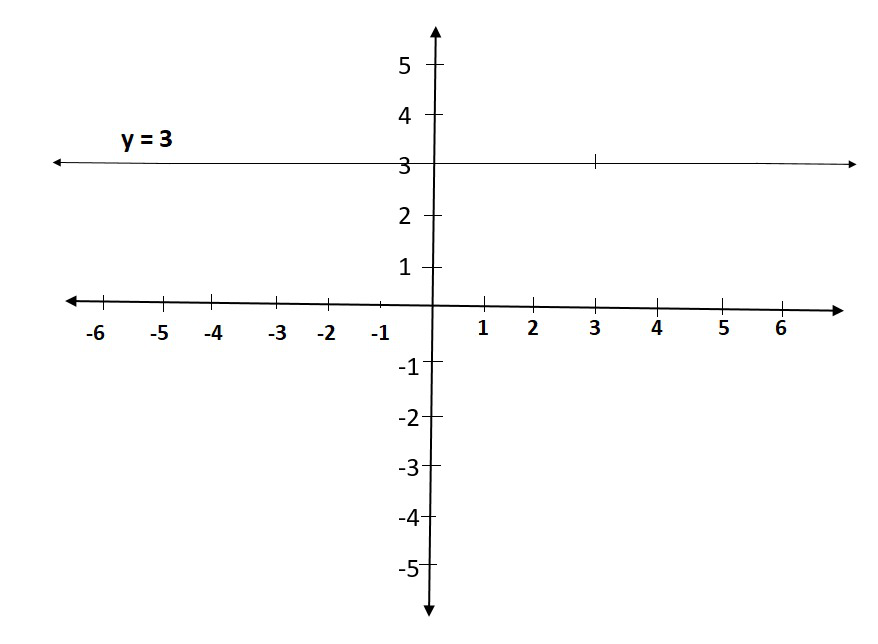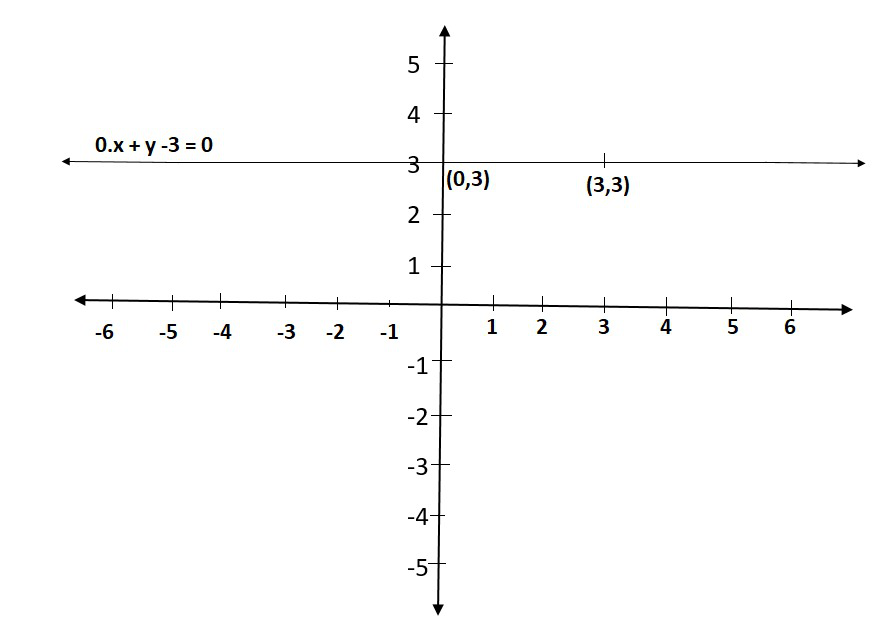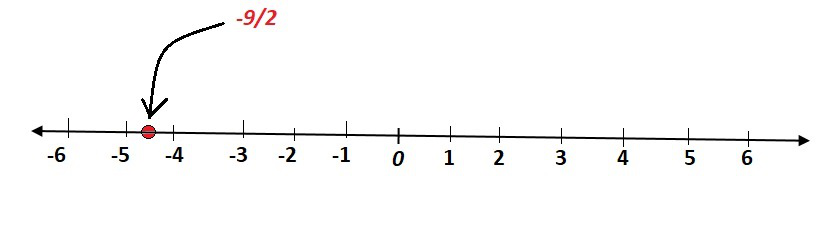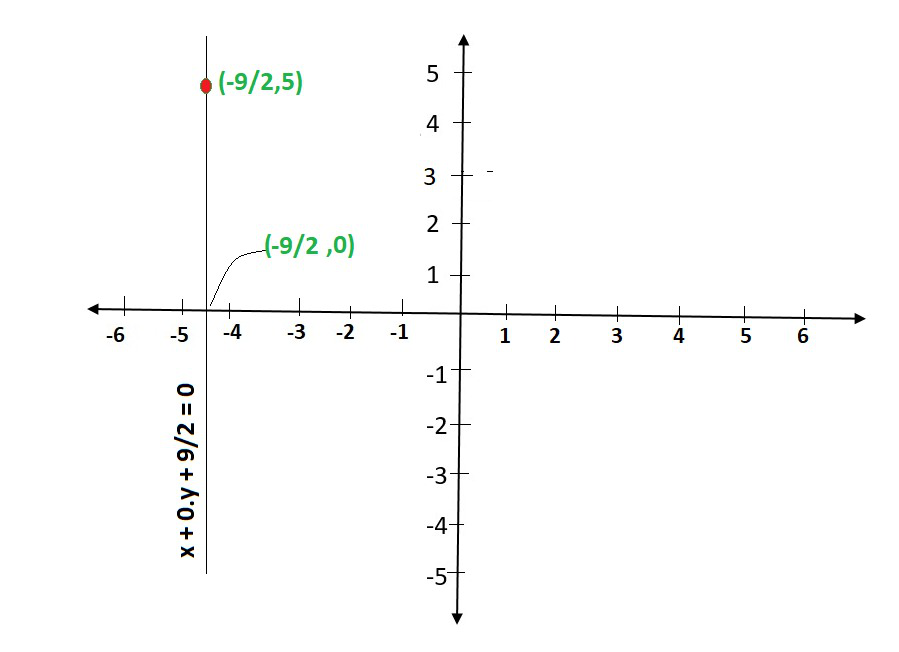Related Articles

# Class 9 NCERT Solutions- Chapter 4 Linear Equations in two variables – Exercise 4.4

• Difficulty Level : Easy
• Last Updated : 03 Jan, 2021

### Question 1. Give the geometric representations of y = 3 as an equation

(i) in one variable

(ii) in two variables

Solution:

(i) If y = 3 is treated as a equation in one variable y only, then it has the unique solution

y = 3, which is a point on the number line(ii) When an equation in two variables, it can be expressed as,

0.x + y – 3 = 0

which is a linear equation in the variables x and y. This is represented by a line. Now all the values of x are permissible because 0.x is always 0. However, y must satisfy the equation y = 3

This has infinitely many solutions. In fact, they are all of the form (r, 3), where r is any real number, so

We can have these two solutions for making line on a graph as:### Question 2. Give the geometric representations of 2x + 9 = 0 as an equation

(i) in one variable

(ii) in two variables

Solution:

Firstly, we solve 2x + 9 = 0, to get

x = -9/2

(i) If x = -9/2 is treated as a equation in one variable x only, then it has the unique solution

x = -9/2, which is a point on the number line.(ii) When an equation in two variables, it can be expressed as,

x + 0.y + 9/2 = 0

which is a linear equation in the variables x and y. This is represented by a line. Now all the values of y are permissible because 0.y is always 0. However, x must satisfy the equation x = -9/2

This has infinitely many solutions. In fact, they are all of the form (-9/2, r), where r is any real number, so

We can have these two solutions for making line on a graph as:Attention reader! All those who say programming isn’t for kids, just haven’t met the right mentors yet. Join the  Demo Class for First Step to Coding Coursespecifically designed for students of class 8 to 12.

The students will get to learn more about the world of programming in these free classes which will definitely help them in making a wise career choice in the future.

My Personal Notes arrow_drop_up